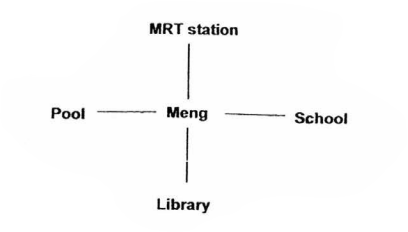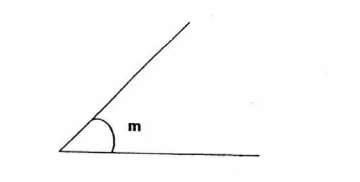# Primary 4 CA1 Practice #1

Time elapsed:
Q1. Which of the following sets of numbers is arranged from the smallest to the greatest?     [2 marks]
Q2. What is twenty thousand and eleven in numerals?     [1 mark]
Q3. Which of the following numbers has the digit 7 in the thousands place?     [1 mark]
Q4. Find the sum of all the factors of 16.     [1 mark]
Q5. In 62 345, the digit 2 stands for _____.     [1 mark]
Q6. Which of the following is a factor of 56?     [1 mark]
Q7. Find the product of 134 and 35.     [1 mark]
Q8. Meng is facing the library. Where will he be facing if he makes a 34 - turn in the clockwise direction?     [1 mark]Q9. Ivan drove 8654 km on trip. Round off the distance travelled to the nearest ten.     [1 mark]
Q10. Which of the following statements is incorrect?     [1 mark]
Q11. Marcus paid \$17 295 for a television set. Round the amount he paid to the nearest thousand dollars.     [1 mark]
Q12. 548 children took part in a colouring competition. Each child was given 30 colour pencils. How many colour pencils were given away?     [1 mark]
Q13. Use a protractor to measure ∠m.     [1 mark]Q14. What is the 6th multiple of 7?     [1 mark]
Q15. Which of the following is a multiple of both 6 and 8?     [2 marks]
Q16. Daryl is thinking of a whole number. It is a factor of 45 and a multiple of 3. What is the number Daryl is thinking of?     [2 marks]
Q17. Which one of the following is the same as 96 141?     [1 mark]
Q18. Find the difference between the 4th multiple of 7 and 6th multiple of 8.     [1 mark]
Q19. What of the following has the same value as 42 326?     [1 mark]
Q20. In 14 507, the digit '1' stands for _____.     [1 mark]